##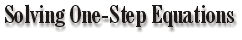An Equation is a mathematical sentence with an equal sign. A Variable is a letter representing a number. x = 3 2 + x = 2 + 3 = 5 x = 8 2x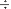4 = 4 164 = 4

To solve one-step equations use the inverse operation.
Addition is the inverse of subtraction and vice versa.
Multiplication is the inverse of division and vice versa.

Variables are used when the equation ( or mathematical sentence) stays the same,
but the numbers (or values) change.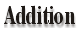Example: x + 4 = -12 The inverse operation is subtraction of 4 from both sides.       x + 4 – 4 = (-12) – 4       x = -16           ?       Check:(-16) + 4 = -12       -12 = -12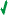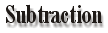Example: d – 8 = -15 The inverse operation is addition of 8 to both sides.       d – 8 + 8 = -15 + 8       d = -7             ?       Check: (-7) – 8 = -15       -15 = -15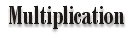Example: -4c = 44 The inverse operation is division of each side by -4.       -4c(-4) = 44(-4)       c = -11           ?       Check: (-4) x (-11) = 44       44 = 44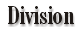Example: F8 = -4 The inverse operation is multiplication of each side by 8.       8 x f8 = (-4) x 8       f = -32           ?       Check: (-32)8 = -4       -4 = -4Example: Jack Nickles is behind in a golftournament. He currently is at – 1 stroke. The leader John Weekly is at – 8 strokes. What score does Jack need on the last hole to win the tournament? Write an equation to represent this problem and then solve it. ANSWER: j + (-1) = -8     The inverse operation is     subtraction of – 1 from     both sides.       j + (-1) – (-1) = (-8) – (-1)       j = -7           ?       Check: (-7) + (-1) = -8       -8 = -8